Function Repository Resource:

# PerturbedCausalGraph

Generate the causal graph produced by combining an unperturbed and perturbed WolframModelEvolutionObject

Contributed by: William Yam
 ResourceFunction["PerturbedCausalGraph"][wm0,wm1] combines the causal graphs of wm0 and wm1 and returns a Graph object.

## Details and Options

ResourceFunction["PerturbedCausalGraph"] tries to find differences between the final state of wm0 and the initial state of wm1 in the form of insertions, deletions and replacements as a way of identifying causally connected edges.
A replacement is considered to be a combination of a deletion and an insertion. The replaced edges do not share the same causal connections as their predecessors.
Edges in the initial state of wm1 that are not contained in the final state of wm0 are assumed to be insertions with no prior causal connections.
The ordering of edges is important and swapping pairs of edges is equivalent to pairs of replacements.
Attempting to combine graphs which share no common edges will result in a disconnected causal graph.

## Examples

### Basic Examples (2)

Compute a WolframModel evolution over 200 steps:

 In:=Out=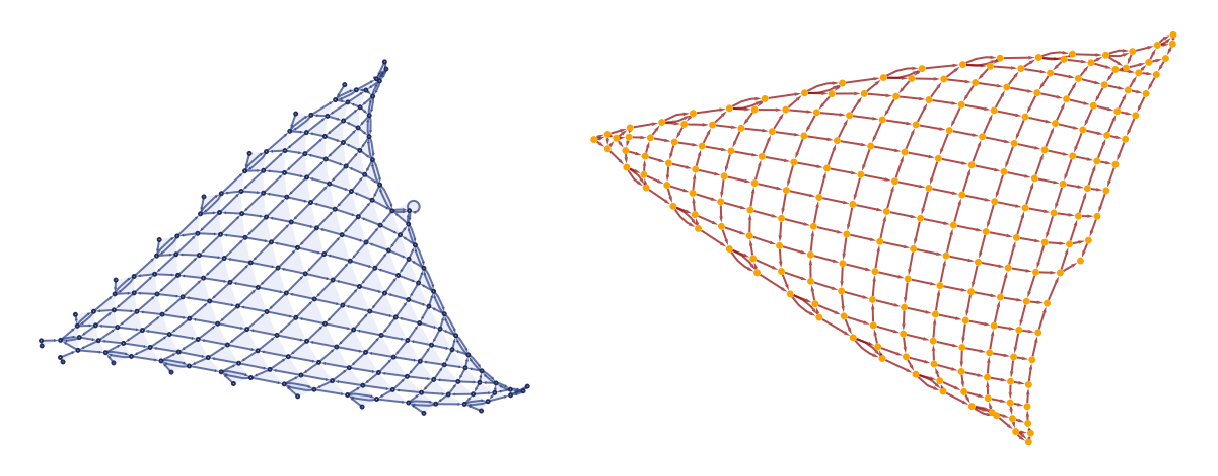After the 200 steps, add a self-loop as a perturbation and evolve for a further 50 steps:

 In:=Out=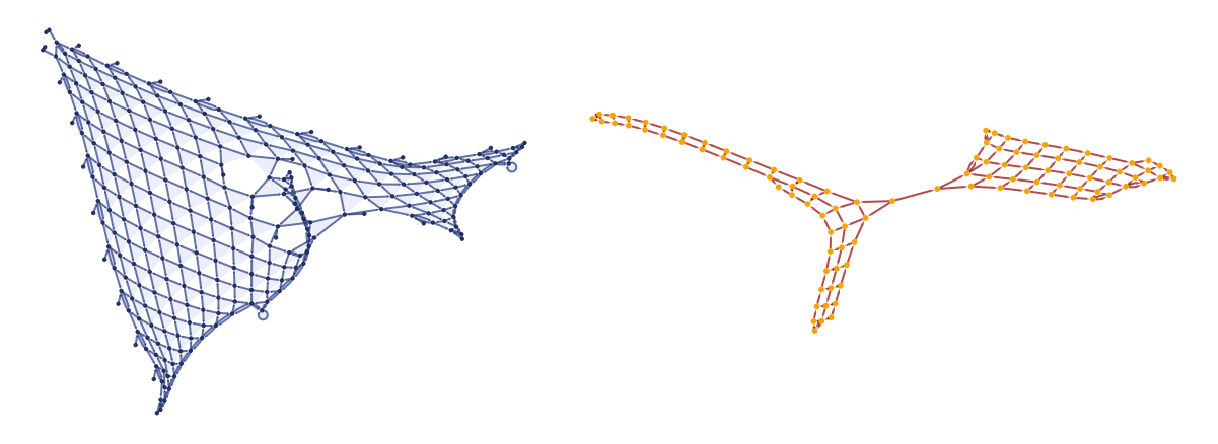Use PerturbedCausalGraph to show the difference caused by the perturbation:

 In:=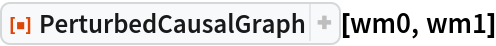Out=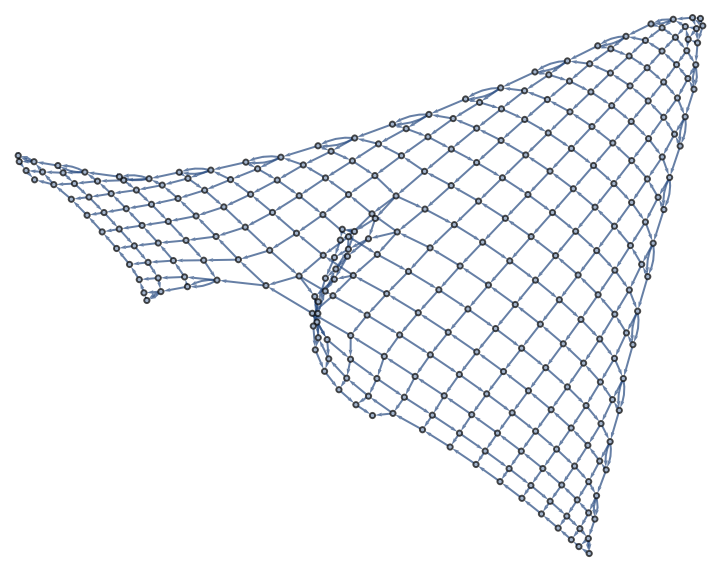Compute 200 steps of the evolution of a WolframModel:

 In:=Plot the final state:

 In:=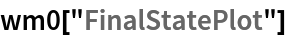Out=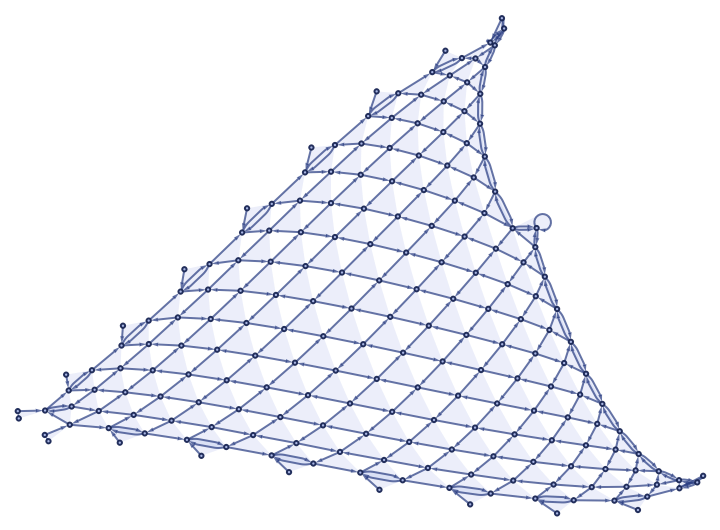Delete 10 random edges in the spatial hypergraph after 200 steps before evolving further:

 In:=In:=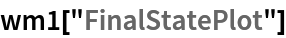Out=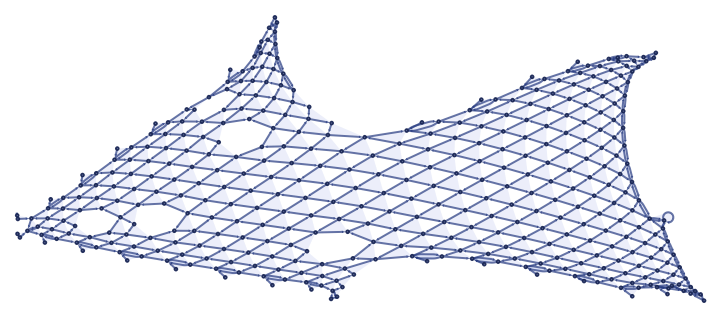Show the perturbed causal graph:

 In:=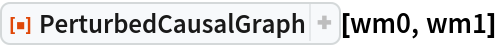Out=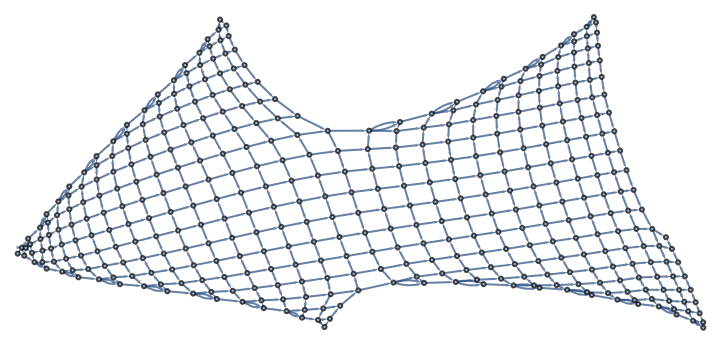### Scope (1)

Use the output of one rule as the input for a different rule:

 In:=Out=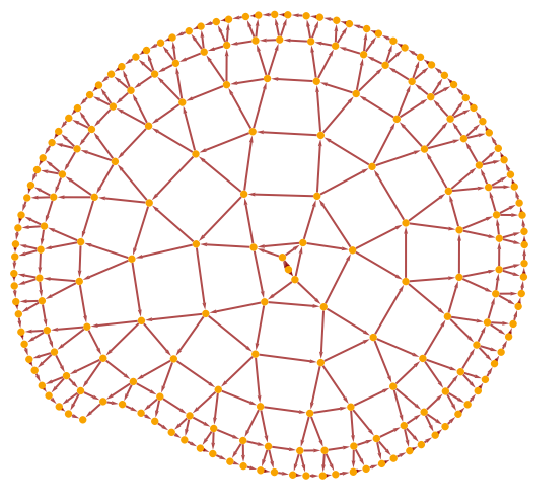In:=In:=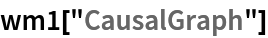Out=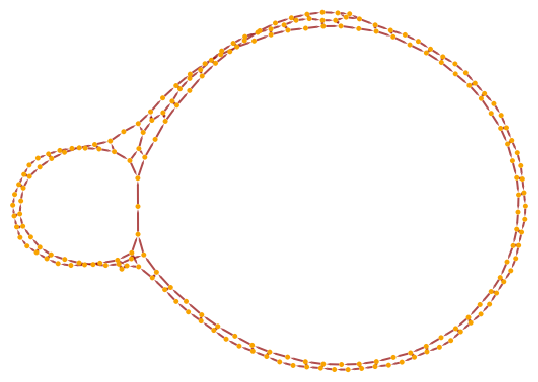In:=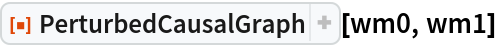Out=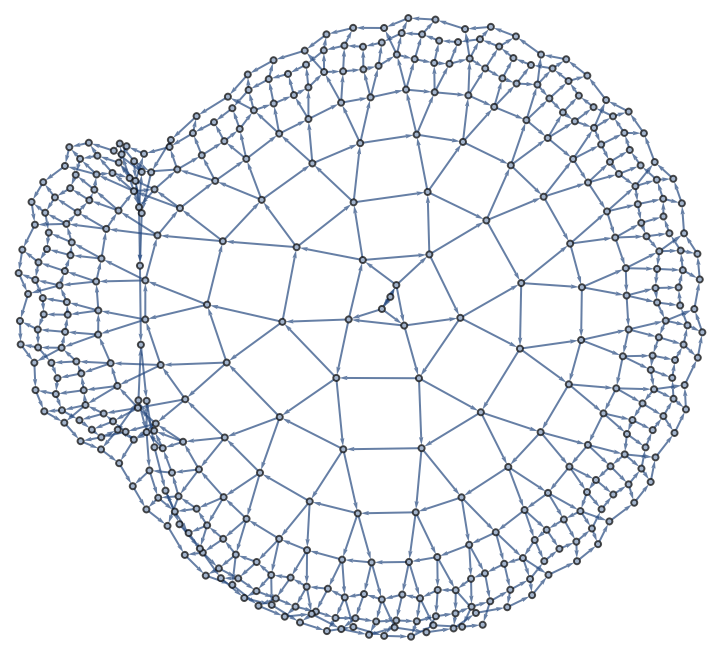## Publisher

Wolfram Summer School

## Version History

• 3.0.0 – 17 September 2020
• 2.0.0 – 17 July 2020
• 1.0.0 – 16 July 2020